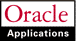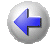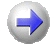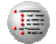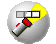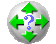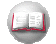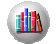Previous Next Contents Index Navigation Glossary Library

## Labor Cost Multipliers

A labor cost multiplier is a value by which Oracle Projects multiplies an employee's labor cost rate to calculate the employee's overtime premium cost rate:

 (Labor Cost Rate) x (Labor Cost Multiplier) = Overtime Premium Labor Cost Rate

Oracle Projects then multiplies this overtime premium labor cost rate by the number of overtime hours an employee works to calculate the overtime premium for that employee:

You define a labor cost multiplier for each kind of overtime your business uses such as double time, or time and a half.

For example, if you pay an employee double time for all overtime hours, you define a labor cost multiplier of 1.0. You multiply the employee's labor cost rate by 1.0 to calculate the employee's overtime premium labor cost rate.

If you pay an employee time and a half for all overtime hours, you define a labor cost multiplier of 0.5 to calculate half the employee's overtime premium labor cost rate.

An employee's total labor cost is the overtime premium plus the total number of hours that employee worked multiplied by the employee's labor cost rate:

(Overtime Premium + Straight Time Labor Cost) = Total Labor Cost

### Defining Labor Cost Multipliers

####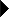To define a labor cost multiplier:

1. In the Labor Cost Multipliers window, enter a unique Name for the labor cost multiplier you are defining. Enter a numeric value for the labor cost multiplier.